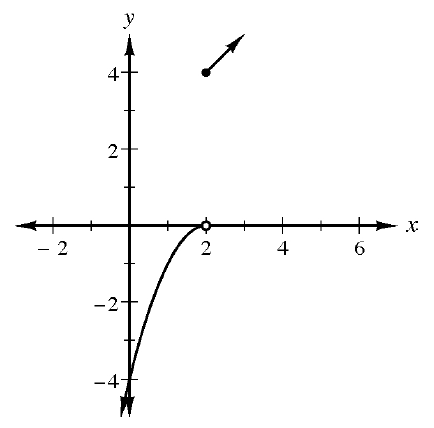Home > CCA2 > Chapter 2 > Lesson 2.2.5 > Problem2-162

2-162.

Write a set of equations for the piecewise-defined function shown on the graph below. Be sure to include the domain for each part of the function. Homework Help ✎Look at the two pieces of the function. One is a downward-facing parabola moved $2$ to the right, and the other is a line.

$f(x)=\begin{cases}-(x-2)^2,x<2\\x+2,x\geq 2 \end{cases}$